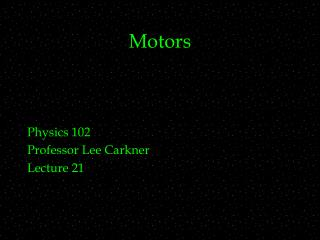DownloadDownload PresentationMotors

# Motors

Download Presentation## Motors

- - - - - - - - - - - - - - - - - - - - - - - - - - - E N D - - - - - - - - - - - - - - - - - - - - - - - - - - -
##### Presentation Transcript

1. Motors Physics 102 Professor Lee Carkner Lecture 21

2. Ring in Solenoid • If the current flows clockwise through the solenoid, the B field inside is straight down • We need to find the flux through the loop before and after the current is switched off • F = BA cos q = BA • B = m0nI = (4pX10-7)(1000)(10) = • A = (0.1)(0.1) =

3. Current in Ring • F = BA = (0.0126)(0.01) = • In 1 second the flux goes to 0 • DF = (1.26 X 10-4) - (0) = 1.26 X 10-4 • Dt = 1 • e = -N(DF/Dt) = (1)(1.26 X 10-4) = • DV = IR or I = e/R = 1.26 X 10-4/10 • I =

4. What is the direction of current in the loop from the PAL (seen from top down)? • clockwise • counterclockwise • left • right • down

5. A bar magnet held north pole up is dropped straight down through a face up coil of wire. What is the direction of the current in the coil as the magnet enters and leaves the coil? • clockwise, counterclockwise • counterclockwise, clockwise • clockwise, clockwise • counterclockwise, counterclockwise • no current is induced

6. Motional emf • If we make the loop larger or smaller, or move it in or out of a field, we will induce a potential • remember emf is a potential difference (or voltage) • How does motion in a field translate to voltage?

7. Consider a conductor of length L sliding on a frame with velocity v but Dx = vDt, so DA = LvDt DF/Dt = BDA/Dt = (BLvDt)/Dt e = BLv Motional emf - Derived DA X B field into page v L x Dx in time Dt

8. Motional emf -- Direction • If the area decreases, the flux decreases and thus the induced B field is in the same direction as the original

9. Generators • What is the most efficient way to use inductance to produce current? • This changing flux produces an emf in the loop • Turn the loop with an external source of work • A machine that converts work into emf is called a generator

10. Alternating Current • Which way does the current flow? • Thus the current flows in one direction and then the other • e.g. household current is at 60 Hz, or 60 cycles per second • This is called alternating current

11. emf From a Generator • Consider a loop of wire rotating in a magnetic field with angular speedw • From Faraday’s Law: • The flux is equal to BA cos q • The change of F with time is thus BAw sin wt, so the emf is: e = NBAw sin wt

12. Sinusoidal Variations emax • As the loop makes one complete rotation (wt goes from 0 to 2p radians) the emf goes from 0, to maximum +, to maximum -, and back to zero again • The current through the loop goes one way and then the other, sometimes is weak and sometimes is strong

13. Frequency • The angular frequency (w) tells you how fast the loop is rotating in radians per second • The number of these cycles made per second is the frequency f = w/2p • The emf equation has two parts • NBAw is the maximum emf

14. Today’s PAL • Consider a generator that consists of a single 1 meter by 1 meter loop of wire with a resistance of 15 W in a magnetic field of 2 T • How many times per second must you rotate the loop to produce a maximum current of 12 amps?

15. Power Generation • Produced (in general) in two ways: • Chemically by batteries • Magnetically by generators • A changing magnetic field separates charges

16. An Alternating Current Generator

17. Motors • If you run a generator backwards it becomes a motor • Motor converts emf to work • As the loop rotates a current is induced in it opposite that of the original • Example: A motor initially has 120 volts, but if the motor produces a back emf of 70 volts, then the total emf is 50 volts

18. Force on Eddy Currents

19. Eddy Currents • Imagine a loop moving out of a magnetic field • As the field through the loop drops, it induces a field in the same direction • If the object is not a loop, circular currents can still be induced which have the same effect • Net effect: • Metal objects moving through a magnetic field will be slowed

20. Next Time • Read 21.7, 21.9-21.11 • Homework: Ch 21, P 14, 23, 30, 39